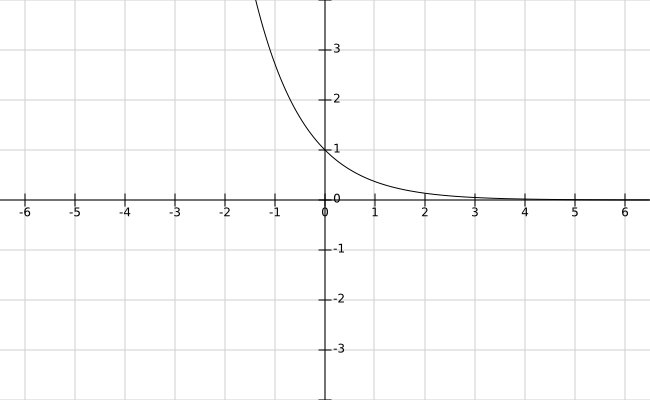# Exponential Function – Graph, Formula, Domain and Range

Here you will learn what is exponential function graph, formula, domain and range.

Let’s begin –

## Exponential Function Formula

Definition : If a is a positive real number other than unity, then a function that associates each x $$\in$$ R to $$a^x$$ is called the exponential function.

In other words, a function f : R $$\rightarrow$$ R defined by f(x) = $$a^x$$, where a > 0 and a $$\ne$$ 1 is called the exponential function.

Formula : It is defined by the formula f(x) = $$a^x$$.

where a > 0 and a $$\ne$$ 1

a is a constant, which is the base of the function

x is any variable.

## Exponential Function Graph

Case 1 : When a > 1,

We observe that the values of y = f(x) = $$a^x$$ increase as the value of x increase.

Also,  f(x) = $$\begin{cases} < 1, & \text{for}\ x > 0 \\ = 1, & \text{for}\ x = 0 \\ > 1, & \text{for}\ x < 0 \end{cases}$$.

Thus, the graph f(x) = $$a^x$$ for a > 1 is  :Case 2 : When 0 < a < 1

In this case, the values of y = f(x) = $$a^x$$ decrease with increase in x and y > 0 for all x $$\in$$ R.

Also, f(x) = $$\begin{cases} > 1, & \text{for}\ x > 0 \\ = 1, & \text{for}\ x = 0 \\ < 1, & \text{for}\ x < 0 \end{cases}$$.

Thus, the graph f(x) = $$a^x$$ for 0 < a < 1 is  :## Domain and Range

We observe that the domain of the exponential function is R the set of all real numbers and the range is the set $$(0, \infty)$$ as it attains only positive values.

Domain : R

Range : $$(0, \infty)$$